# HSSlive: Plus One & Plus Two Notes & Solutions for Kerala State Board

## AP Board Class 7 Maths Chapter 1 Integers Ex 1 Textbook Solutions PDF: Download Andhra Pradesh Board STD 7th Maths Chapter 1 Integers Ex 1 Book AnswersAP Board Class 7 Maths Chapter 1 Integers Ex 1 Textbook Solutions PDF: Download Andhra Pradesh Board STD 7th Maths Chapter 1 Integers Ex 1 Book Answers

## Andhra Pradesh State Board Class 7th Maths Chapter 1 Integers Ex 1 Books Solutions

 Board AP Board Materials Textbook Solutions/Guide Format DOC/PDF Class 7th Subject Maths Chapters Maths Chapter 1 Integers Ex 1 Provider Hsslive

2. Click on the Andhra Pradesh Board Class 7th Maths Chapter 1 Integers Ex 1 Answers.
3. Look for your Andhra Pradesh Board STD 7th Maths Chapter 1 Integers Ex 1 Textbooks PDF.

## AP Board Class 7th Maths Chapter 1 Integers Ex 1 Textbooks Solutions with Answer PDF Download

Find below the list of all AP Board Class 7th Maths Chapter 1 Integers Ex 1 Textbook Solutions for PDF’s for you to download and prepare for the upcoming exams:

Question 1.
Some integers are marked on the number line. Which is the biggest and which is the
smallest?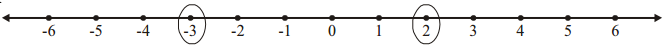Solution:
The numbers marked = -3 and 2
biggest number = 2
smallest number = -3

Question 2.
Write the integers between the pairs of integers given below. Also, choose the biggest and
smallest intergers from them.
(i) – 5, – 10
(ii) 3, – 2
(iii) – 8, 5
Solution:
i) – 5, – 10
Integers between – 5, – 10 are – 6, – 7, – 8, – 9
smallest Integer – 9
biggest integer = – 6

ii) 3, – 2
Integers between 3 and – 2 are – 1,0, 1, 2
smallest integer = – 1
biggest integer 2

iii) – 8, 5
Integers between – Sand 5 are 4, 3, 2, 1, 0, – 1, – 2, – 3, – 4, – 5, – 6, – 7
smallest integer = – 7
biggest integer = 4

Question 3.
Write the following integers in ascending order (smallest to biggest).
(i) – 5, 2, 1, – 8
(ii) – 4, – 3, – 5, 2
(iii) – 10, – 15, – 7
Solution:
(i) – 8. – 5, 1, 2
(ii) – 5, – 4, – 3, 2
(iii) – 15, – 10, – 7

Question 4.
Write the following integers in descending order (biggest to smallest).
(i) – 2, – 3, – 5
(ii) – 8, – 2, – 1
(iii) 5, 8, – 2
Solution:
(i) – 2, – 3, – 5
(ii) – 1, – 2, – 8
(iii) 8, 5, – 2

Question 5.
Represent 6, – 4, 0 and 4 on a number line.
Solution: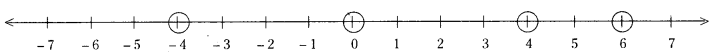Question 6.
Fill the missing integers on the number line given below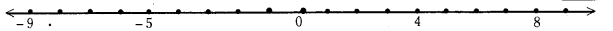Solution: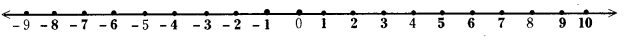Question 7.
The temperatures of 5 cities in india on a particular day are shown on the number line below.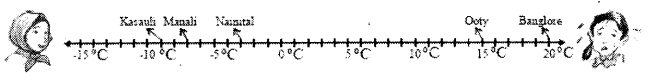(i) Write the temperatures of the cities marked on it?
(ii) Which city has the highest temperature?
(iii) Which city has the lowest temperature?
(iv) Which cities have temperature less than 0°C?
(v) Name the cities with temperature more than 0°C?
Solution:
i) The temperatures of the cities shown on the nL.lber line an
Kasauli
Manali = – 7°C
Nainital = – 3°C
Ooty = 15°C
l3anglore = 20°C

ii) The city with highest temperature is Banglore.
iii) The city with lowest temperature is Kasauli.
iv) The cities with temperatures less than 0°C are Nainital, Manali and Kasauli.
v) The cities with temperatures more than 0°C are Ooty and Banglore

## Andhra Pradesh Board Class 7th Maths Chapter 1 Integers Ex 1 Textbooks for Exam Preparations

Andhra Pradesh Board Class 7th Maths Chapter 1 Integers Ex 1 Textbook Solutions can be of great help in your Andhra Pradesh Board Class 7th Maths Chapter 1 Integers Ex 1 exam preparation. The AP Board STD 7th Maths Chapter 1 Integers Ex 1 Textbooks study material, used with the English medium textbooks, can help you complete the entire Class 7th Maths Chapter 1 Integers Ex 1 Books State Board syllabus with maximum efficiency.

## FAQs Regarding Andhra Pradesh Board Class 7th Maths Chapter 1 Integers Ex 1 Textbook Solutions

#### Can we get a Andhra Pradesh State Board Book PDF for all Classes?

Yes you can get Andhra Pradesh Board Text Book PDF for all classes using the links provided in the above article.

## Important Terms

Andhra Pradesh Board Class 7th Maths Chapter 1 Integers Ex 1, AP Board Class 7th Maths Chapter 1 Integers Ex 1 Textbooks, Andhra Pradesh State Board Class 7th Maths Chapter 1 Integers Ex 1, Andhra Pradesh State Board Class 7th Maths Chapter 1 Integers Ex 1 Textbook solutions, AP Board Class 7th Maths Chapter 1 Integers Ex 1 Textbooks Solutions, Andhra Pradesh Board STD 7th Maths Chapter 1 Integers Ex 1, AP Board STD 7th Maths Chapter 1 Integers Ex 1 Textbooks, Andhra Pradesh State Board STD 7th Maths Chapter 1 Integers Ex 1, Andhra Pradesh State Board STD 7th Maths Chapter 1 Integers Ex 1 Textbook solutions, AP Board STD 7th Maths Chapter 1 Integers Ex 1 Textbooks Solutions,
Share: